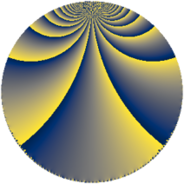# Properties

 Label 1344.4.wLevel $1344$ Weight $4$ Character orbit 1344.w Rep. character $\chi_{1344}(337,\cdot)$ Character field $\Q(\zeta_{4})$ Dimension $144$ Sturm bound $1024$

# Related objects

## Defining parameters

 Level: $$N$$ $$=$$ $$1344 = 2^{6} \cdot 3 \cdot 7$$ Weight: $$k$$ $$=$$ $$4$$ Character orbit: $$[\chi]$$ $$=$$ 1344.w (of order $$4$$ and degree $$2$$) Character conductor: $$\operatorname{cond}(\chi)$$ $$=$$ $$16$$ Character field: $$\Q(i)$$ Sturm bound: $$1024$$

## Dimensions

The following table gives the dimensions of various subspaces of $$M_{4}(1344, [\chi])$$.

Total New Old
Modular forms 1568 144 1424
Cusp forms 1504 144 1360
Eisenstein series 64 0 64

## Trace form

 $$144q + O(q^{10})$$ $$144q - 40q^{11} + 240q^{15} + 48q^{19} - 400q^{29} - 16q^{37} + 1672q^{43} - 7056q^{49} + 1488q^{51} - 752q^{53} + 1824q^{61} - 504q^{63} - 1952q^{65} - 408q^{67} + 1056q^{69} + 2208q^{75} - 1904q^{77} + 11984q^{79} - 11664q^{81} + 5360q^{83} + 480q^{85} - 360q^{99} + O(q^{100})$$

## Decomposition of $$S_{4}^{\mathrm{new}}(1344, [\chi])$$ into newform subspaces

The newforms in this space have not yet been added to the LMFDB.

## Decomposition of $$S_{4}^{\mathrm{old}}(1344, [\chi])$$ into lower level spaces

$$S_{4}^{\mathrm{old}}(1344, [\chi]) \cong$$ $$S_{4}^{\mathrm{new}}(16, [\chi])$$$$^{\oplus 12}$$$$\oplus$$$$S_{4}^{\mathrm{new}}(48, [\chi])$$$$^{\oplus 6}$$$$\oplus$$$$S_{4}^{\mathrm{new}}(64, [\chi])$$$$^{\oplus 4}$$$$\oplus$$$$S_{4}^{\mathrm{new}}(112, [\chi])$$$$^{\oplus 6}$$$$\oplus$$$$S_{4}^{\mathrm{new}}(192, [\chi])$$$$^{\oplus 2}$$$$\oplus$$$$S_{4}^{\mathrm{new}}(336, [\chi])$$$$^{\oplus 3}$$$$\oplus$$$$S_{4}^{\mathrm{new}}(448, [\chi])$$$$^{\oplus 2}$$